Online Tution   »   CBSE Class 10 Answer Key (All...   »   CBSE Class 10 Maths Term 2...

# CBSE Class 10th Maths Term 2 Answer Key and Paper Solution (Standard and Basic)

## Class 10 Maths Term 2 Answer Key 2022

The Central Board of Secondary Education is all set to conduct Class 10 Term 2 Mathematics Exam 2022 on 5th May 2022. After examination, the students will rush to find CBSE Class 10 Term 2 Mathematics Answer key 2022 to cross check their responses. We have provided the CBSE Class 10 Term 2 Mathematics Answer key 2022 on this page. The CBSE Class 10 Term 2 Mathematics Answer key 2022 is prepared by the expert facilities of Adda247. The Students appearing in the CBSE Term 2 Exam 2022 must bookmark this page to get CBSE Term 2 Answer Keys of other subjects.

Check Here: CBSE Class 10 Science Term 2 Answer key 2022

Check Out: CBSE Class 10 English Term 2 Answer Key

## CBSE Class 10 Maths Term 2 Answer Key

With the help of CBSE Class 10 Term 2 Mathematics Answer key 2022, the students can cross check their responses. CBSE Class 10 Term 2 Mathematics Answer key 2022 is prepared by experienced faculties and it’s error free, so candidates can match their responses with the CBSE Class 10 Term 2 Mathematics Answer key 2022 provided on this page. Go through whole article

## CBSE Class 10th Maths Answer key Term 2

The students appearing in the CBSE Class 10 Term 2 Exam 2022 must know the miscellaneous information regarding CBSE Class 10th Answer key Mathematics Term 2 provided in the table below:

 Class 10 Mathematics Term 2 Answer Key 2022 Exam Conducting Body Central Board of Secondary Education Exam & Subject Name CBSE Class 10 Mathematics Category Class 10 Maths Term 2 Answer Key 2022 Exam Date 5th May 2022 Unofficial Answer Key 5th May 2022 Official Answer Key To be notified Official Website https://www.cbse.nic.in/

## Class 10 Maths Term 2 Answer Key 2022: Question Paper Pattern

The exam pattern is based on the CBSE Class 10 Term 2 Mathematics Sample Paper released by the board on its official website. As per the CBSE Class 10 Term 2 Mathematics Sample Paper, the Class 10 Mathematics question paper consists of 3 sections namely, Section A, Section B, Section C. The details of these sections are:

Section A: In this section, 6 questions will be asked. Each question carries 2 marks.

Section B: In this section, 4 questions will be asked. Each question carries 3 marks.

Section C: In this section, 4 questions will be asked. Each question carries 4 marks.

Standard-

Basic

## CBSE Class 10 Term 2 Mathematics Standard Solution: Paper Code-30/1/1, Set 1

Section A

Q.1 Find the sum of first 30 terms of AP: -30, 24, -18,/..

Solution: a= -30, d= 6

= 15 [-60 + 29 (6)]

= 15 [-60+174]

= 15 

= 1710

OR

Q:  If an AP is Sn = n(4n+1), then find the AP

Solution: S1 = T1 = 5

S2= T1 + T2= 18

S2-S1 = T2= 13

AP= 5, 13, 21, 29, …

Q.2: A solid metallic sphere of radius 10.5 cm is melted and recast into a number of smaller cones, each of radius 3.5 cm and height 3 cm. Find the number of cones so formed.

Solution: r1 = 10.5 cm (sphere) and r2 (cone)= 3.5 cm, h2= 3cm

4/3πr³ = n x 1/3πr2² h

4r³ = nr2² h

4 x (105/10)³= n x 35/10 x 35/10 x 3

21 x 6= n

Number of cones formed= 126

Q. 3 (a) Find the value of m for which the quadratic equation

(m-1) x² + 2(m-1)x + 1 = 0

Solution: D= 0

4 (m-1)² – 4(m-1) = 0

4 (m-1) [m-1-1]= 0

(m-1) (m-2)= 0

m= 2

(b) Solve the following quadratic equation for x: √3x² + 10x + 7√3= 0

Solution: √3x² + 3x + 7x + 7√3= 0

√3x (x+ √3) + 7 (x + √3)= 0

(x+ √3) [√3x + 7]= 0

x= √3 || x= -7/√3

Q.4: Find the mode of the following frequency distribution:

 Class 10-20 20-30 30-40 40-50 50-60 Frequency 15 10 12 17 4

Solution: l= 40, f1= 17, f2= 4, fo= 12

Mode= l + f1-fo / 2f1 – fo- f2

= 40 + 17-12 / 34-12-4 x 10

= 40 + 5/18 x 10

= 40 + 25/9

= 40 + 2.78

= 42.78

Q.5: The product of Rehan’s age (in years) 5 years ago and his age 7 years from now, is one more than twice his present age. Find his present age.

Solution:

 Past Age Present Age Future Age x-5 x x+7

(x-5) (x+7) = 2x + 1

x² + 2x -35 = 2x+1

x² – 36 = 0

x² = 36

x= +_ 6

Present Age- 6 years

Q.6 Two concentric circles are of radii 4 cm and 3 cm. Find the length of the chord of the larger circle which touches the smaller circle.

Solution: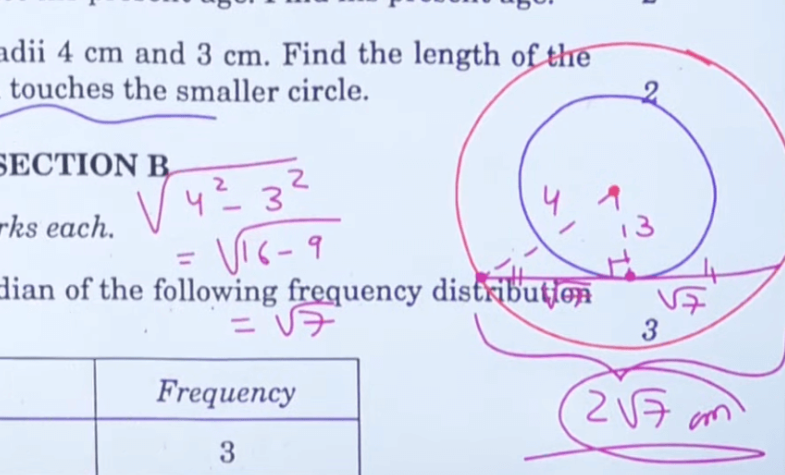Section B

Q.7: For what value of x, is the median of the following frequency distribution 34:5?

 Class Frequency 0-10 3 10-20 5 20-30 11 30-40 10 40-50 x 50-60 3 60-70 2

Solution: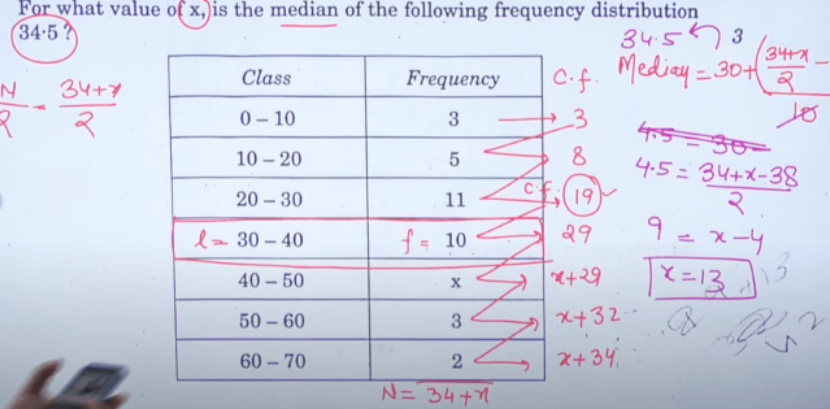Q.8: Draw a circle of radius 3 cm. Take two points P and Q on one of its extended diameter each at a distance of 7 cm from its centre. Construct tangents to the circle from these two points P and Q.

Solution: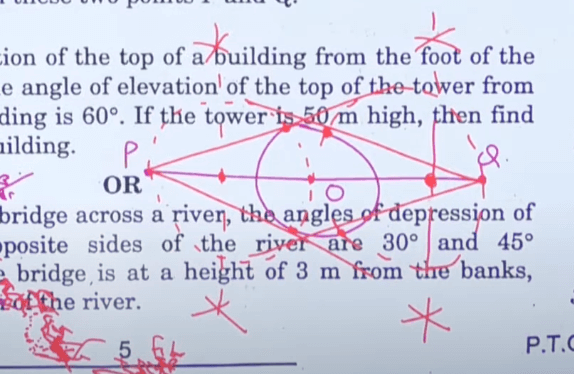Q.9: (a) The angle of elevation of the top of a building from the foot of the tower is 30° and the angle of elevation of the top of the tower from the foot of the building is 60°. If the tower is 50 m high, then find the height of the building.

Solution: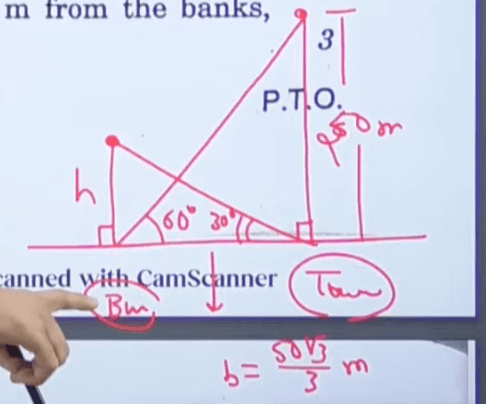tan 60° = 50/b

b= 50/ tan 60°

b = 50/ √3

b= 50√3 / 3

tan 30° = h/(50√3/3)

h= 50√3/3 x 1/√3

= 50/3 m= 16.67 metres

OR

Q.9:(b) From a point on a bridge across a river, the angles of depression of the banks on opposite sides of the river are 30° and 45° respectively. If the bridge is at a height of 3 m from the banks,  then find the width of the river.

Solution: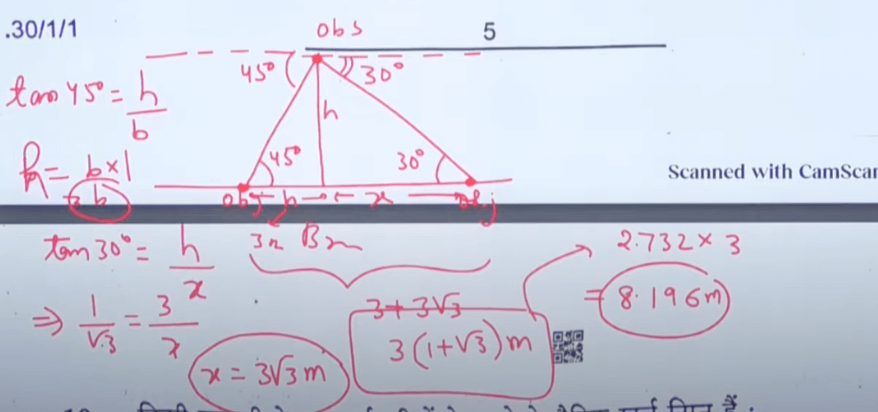Q.10: Following is the daily expenditure on lunch by 30 employees of a company, Find the mean daily expenditure of the employees.

Solution: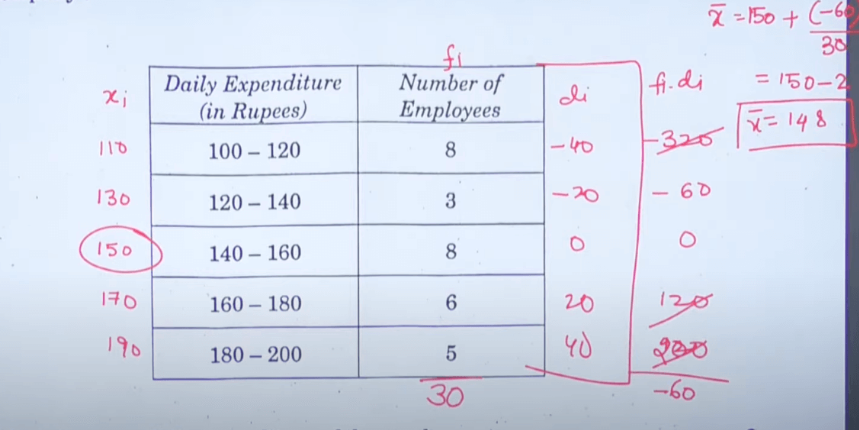Q.11: From a solid cylinder of the height of 30 cm and radius of 7 cm, a conical cavity of height of 24 cm and same radius is hollowed out. Find the total surface area of the remaining solid.

Solution: H= 30 cm

R= 7 cm

Total Surface Area of Cylinder = Curved Surface Area of Cylinder + Curved Surface Area of Cone + πr²

TSA = 2 πrh +  πrl + πr²

= πr (2h+ l+ r)

= 22/7 x 7 (60+25 + 7)

= 22 x 92

TSA= 2024 cm

Q.12: In figure 1, a traingle ABC with angle B = 90 degrees is shown. Taking AB as diameter, a circle has been drawn intersecting AC at point P. Prove that the tangent drawn at point P bisects BC.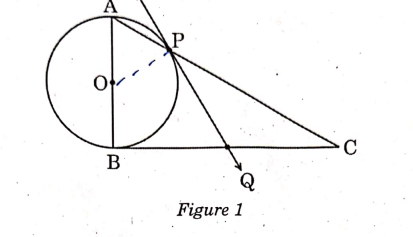Solution: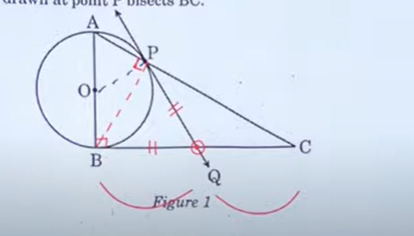Case Study:1

Q.13: In Mathematics, relations can be expressed in various ways. The matchstick patterns are based on linear relations. Different strategies can be used to calculate the number of matchsticks used in different figures.

One such pattern is shown below. Observe the pattern and answer the following questions using Arithmetic Progression::

(a)  Write the AP for the number of triangles used in the figures. Also, write the nth term of this AP. Which figure has 61 matchsticks?

Solution- AP = 4, 6, 8, 10

a= 4, d= 2

Tn = 4 + 2(n-1)

Tn= = 4 + 2n – 2

Tn= 2n +2

(b) Which figures has 61 matchstickes?

Solution: 12, 19, 26, …

Tn = 61

a + (n-1) d= 61

12 + 7(n-1) = 61

7(n-1) = 49

n-1 = 7

n= 8

8th figure has 61 matchsticks

Q.14 Gadisagar lake is located in the jaisalmer district of Rajasthan. It was built by king of jaisalmer ad rebuilt by Gadsi Singh in 14th Century. The lake has many chattris. One of them is shown below: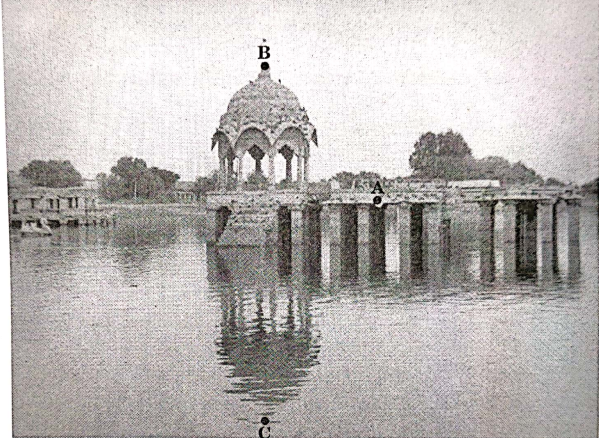Observe the picture. From a point A h m above from water level, the angle of elevation of top of chattari (point B) is 45 degrees and angle of depression of its reflection in water(Point C) is 60 degrees. If the height of chhattri above the water level is 10m, then

a) Draw a well-labelled figure based on the above information;

b) Find the height of the point A above the water level.

(Use √3 = 1.73)

Solution: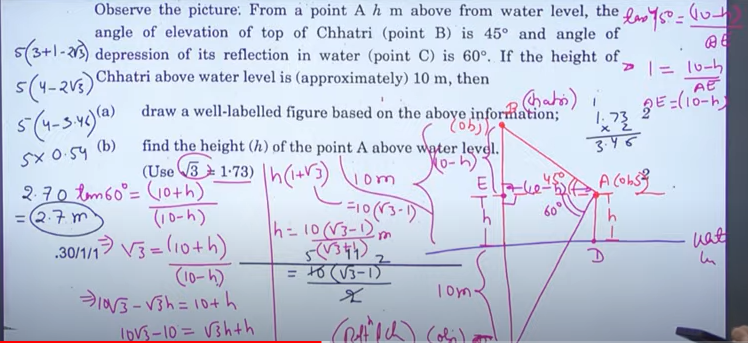Check Out: Answer keys of Term 1 And Term 2

## CBSE Class 10th Maths Term 2 Answer Key 2022: FAQs

Q. Where can I get the Class 10 Mathematics Term 2 Answer Key 2022?

Q. How many marks does the Term 2 Mathematics examination in Class 10th carry?

The Class 10 Mathematics Term 2 examination carries 40 marks.

Q. When will the CBSE hold the Term 2 Mathematics paper for students in Class 10th?

CBSE will hold the Class 10 Mathematics Paper for Term 2 on the 5th May 2022.

Q. Will CBSE release the official answer key of Class 10 Term 2 Mathematics?

The CBSE has not informed yet about the CBSE Class 10 Term 2 Answer key. When CBSE releases the official Class 10th Term 2 Mathematics Answer Key 2022, we will update the same here.

Sharing is caring!

Thank You, Your details have been submitted we will get back to you.